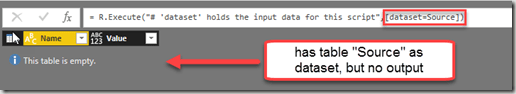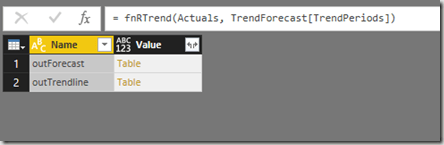# Tips and Tricks for R scripts in the query editor in Power BI

Especially if you are new to R, there are some things one needs to know to successfully run R-scripts in the query editor of Power BI. I will share them here along with some tricks that made my R-life in Power BI easier:

### You can feed multiple tables into the R-script

If you click the icon “R script”, the table from the previous step will automatically be passed as the “dataset” to the R-script. So if you don’t fill in any R-code, this will happen:But if you need the content from other tables as well, you just add them into the square brackets like this:“Documentation” looks like this:### You can use parameters in the R-script

Apart from tables, you can also use text strings as parameters in the script. They need to be inserted into the code with preceeding “& and trailing &”:

RExportCsv= R.Execute(“write.csv(dataset,” “&CsvExportPath&” “)”,[dataset=Actuals])

Beware that they must be text. So if you want to pass a number, wrap it into Text.From(…).

### You cannot use anything else apart from tables and parameters in the R-script

Well, at least I haven’t managed it### R-life gets easy-peasy if you use M-functions for your R-script

For an R-script like export to csv for example, you have to reverse all slashes in your path-definition. This is a bit tedious. So just create an M-function that reverses them automatically (row 4) before passing to the R-script like this:

This file contains bidirectional Unicode text that may be interpreted or compiled differently than what appears below. To review, open the file in an editor that reveals hidden Unicode characters.

 // Exports the current table to csv. Pass full PathFile specification as parameter (path as text, dataset as table) => let ConvertedPath = Text.Replace(path, "\", "/"), RScript = R.Execute("write.csv(dataset,"""&ConvertedPath&""") output <- dataset ",[dataset=dataset]), output = RScript{[Name="output"]}[Value] in output

view raw

ExportCsv.pq

hosted with ❤ by GitHub

Another advantage: You can create a nice user-dialog by adjusting the function metadata like this for example:So the user doesn’t have to see that R-code is used here.

This file contains bidirectional Unicode text that may be interpreted or compiled differently than what appears below. To review, open the file in an editor that reveals hidden Unicode characters.

 let func = // fnStat.Trend // Author: Imke Feldmann – http://www.thebiccountant.com/ – Link to blogpost: http://wp.me/p6lgsG-Fd (Actuals as table, FCPeriods as list) => let FCPeriods = Table.FromList(FCPeriods, Splitter.SplitByNothing()), #"Run R Script" = R.Execute("y <- as.matrix(Actuals) x <- as.matrix(Actuals) trendline <- fitted(lm(y ~ x)) forecast <- predict(lm(y ~ x), list(x = as.matrix(FCPeriods))) outTrendline <- cbind(Actuals, data.frame(trendline)) outForecast <- cbind(FCPeriods, data.frame(forecast))" ,[Actuals=Actuals, FCPeriods=FCPeriods]), RStatTrend = #"Run R Script" in RStatTrend , documentation = [ Documentation.Name = " fnRStat.Trend ", Documentation.Description = " R-function for trend (fitted(lm) and forecast (predict(lm) " , Documentation.LongDescription = " R-function for trend (fitted(lm) and forecast (predict(lm) ", Documentation.Category = " R Statistical Functions ", Documentation.Source = " local ", Documentation.Author = " Imke Feldmann: www.TheBIccountant.com ", Documentation.Examples = {[Description = " 1) Choose table with actual figures 2) choose column holding the forecast periods " , Code = " Check this blogpost explaining how it works: http://wp.me/p6lgsG-Fd ", Result = " "]}] in Value.ReplaceType(func, Value.ReplaceMetadata(Value.Type(func), documentation))

view raw

R Trend.pq

hosted with ❤ by GitHub

### Output of the R-script has to be a data-frame

This one has fooled me a bit: My script worked fine in RStudio, but didn’t return any results in Power BI. As it turned out, the result of the script was a list (or vector) and not a dataframe. So I had to convert it to a dataframe (see row 12 & 13 in R Trend m) and then it worked alright.

### You need to assign desired outputs

This might also not be too obvious for beginners: You can successfully run R-code, but not get any result returned from your R-script: Check the ExportCsv-script above: It would export the table to the desired path without the code in row 6 (“output <- dataset”), but we wouldn’t be able to see the data or further work on it in the query editor. So if you use the R-script for transformations and then further work on the results or load them to the data model, you need to create an output by assigning via the “<-“.

### You can have multiple output entities

If you run the trend-function from above, you will get a table like this back:outTrendline contains the trendline for the actual figures and outForecast the trend-predictions for the future periods (…the assignments from row 8-11 are not returned here, because they are not in dataframe-format).

I hope this will help getting you started quickly with R in M !

Do you agree that it’s a real pity that we don’t have the R-integration in Power Query in Excel (yet?)?. Then please

Enjoy & stay queryious 🙂

### Comments (11) Write a comment

1.I really like your articles, and was thrilled when I saw this. But I just can’t seem to get it to work. I’ve tried to do exactly like in the example, but when I try to load two datasets like this [dataset=Source, dataset2=tbl_A] where Source is the last loaded table and tbl_A is another independent table, it just raises a Formula.Firewall error. Could you provide any more details with regards to how you’ve built your example? Thanks!

•Hi Arnie,
this is a general issue with R-scripts and external data sources: You need to adjust your privacy settings to always ignore privacy settings.
Thanks for bringing this up, should have mentioned it in the post!

2.How do I call this export to csv function for each row table with a path_to_csv column and a list of table’s column ? Would each line export an csv file? I want to “invoke Custom Funcion” passing columns as parameters.

(path as text, dataset as table) =>
let
ConvertedPath = Text.Replace(path, “\”, “/”),
RScript = R.Execute(“write.csv(dataset,”””&ConvertedPath&”””)
output <- dataset
“,[dataset=dataset]),
output = RScript{[Name=”output”]}[Value]
in
output

•Yes, if you call this function in a custom column, for each row 1 csv-file would be created.

3.Hi Imke,
One question, I’m using an R script in Power BI (Power Query/Transform) to cluster customers according to their historical transactions.
My script looks like this:
library(cluster)
k.means.fit_log <- kmeans(dataset[2:4], 3)
output<-dataset
output\$cluster <- k.means.fit_log\$cluster
This adds a column to the table with the custer number. It’s fine, but the output of the custer algorithm is k.means.fit_log and it contains a lot more information I’d like to analyse, like custer centers, size, etc.
In R k.means.fit_log is a list of 9, so I’d like to create 9 tables in Power BI with all these details.
How can I do this? Any idea?
Thank you so much for your help and keep writing excellent stuff!

•If my understanding is correct you can use the result of the R-script as a staging-table in the query editor that you don’t load.
Then reference this table from your new tables where you pick out one result and load it as a dedicated table.
/Imke

•Hard to say. The table is returned, so the R-script has run without an error. Could it be an issue with your input data?
How about creating a “hardcoded table” as a test-input for your script?

•4.